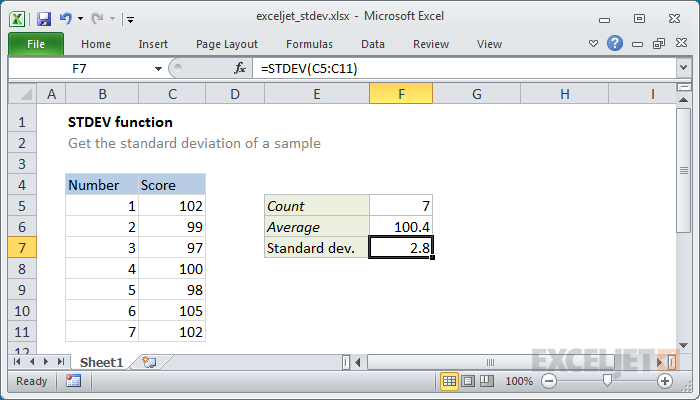# This tutorial shows how to calculate the standard deviation based on the total population using the STDEV.P function in Excel and how to estimate the standard deviation based on the sample using the STDEV.S function in Excel.

## What is standard deviation?

Deviation is a numerical measure that says how far the numbers are from the mean.

1. For example, numbers below the average have an average of 10.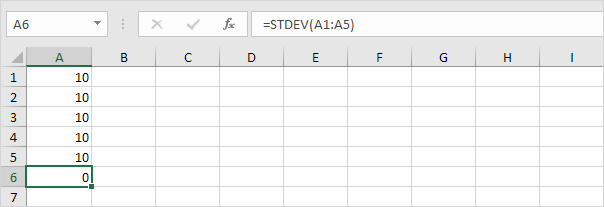Explanation: The numbers are all the same and this means that there is no change. As a result, the numbers have a standard deviation of zero. The STDEV function is an old function. Microsoft Excel recommends using the new STEDV.S function, which produces exactly the same result.

2- The following numbers also have an average (average) of 10.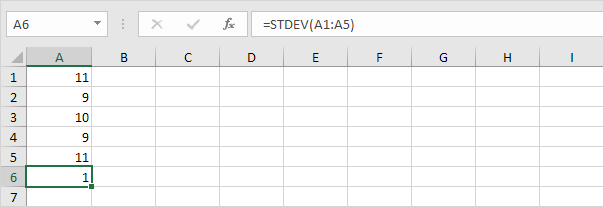Explanation: The numbers are close to the mean. As a result, they have low standard deviations.

3- The following numbers also have an average of 10.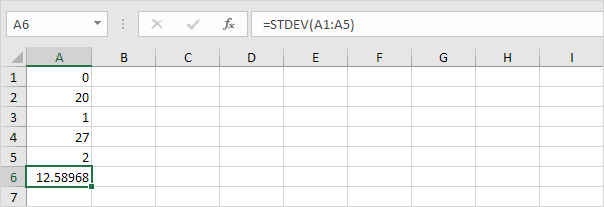Explanation: The numbers are spread. As a result, they have a high standard deviation.

## STDEV.P

The STDEV.P (P stands for Population) function in Excel calculates the standard deviation based on the total population. For example, you are teaching 5 students. You have test scores for all students. The total population consists of 5 data points. The STDEV.P function uses the following formula: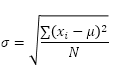In this example x1 = 5, x2 = 1, x3 = 4, x4 = 6, x5 = 9, μ = 5 (mean), N = 5.

1- Calculating the mean (μ).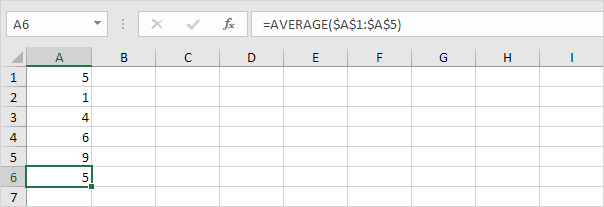2- Calculate the distance to the average for each number.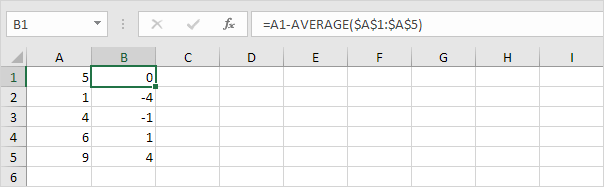3- Calculate the second power of the distance for each number.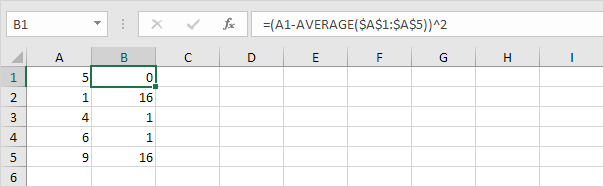4- Sum of values ​​(1).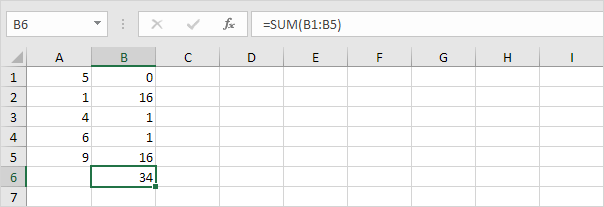5- Divide by the number of data points (N = 5).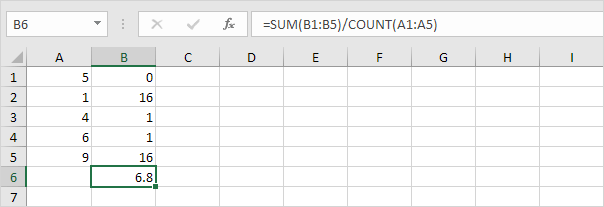6- Calculating the second root of the obtained number.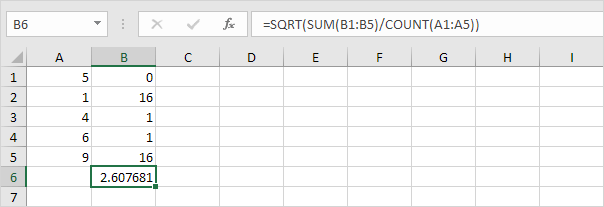7- Fortunately, the STDEV.P function in Excel can do all these steps for you.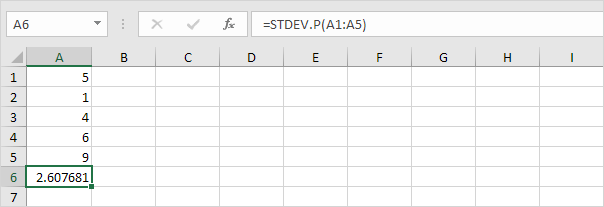## STDEV.S

The STDEV.S function (S stands for Sample) in Excel estimates the standard deviation based on the sample. For example, you are teaching a large group of students but only have the test scores of 5 students. The sample size is 5. The STDEV.S function uses the following formula: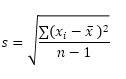In this example (same numbers as above) x1 = 5, x2 = 1, x3 = 4, x4 = 6, x5 = 9, (sample mean) 5 = x̄, n = 5 (sample size).

1- Repeat steps 1 to 5 above, but in step 5, divide by n-1 instead of N.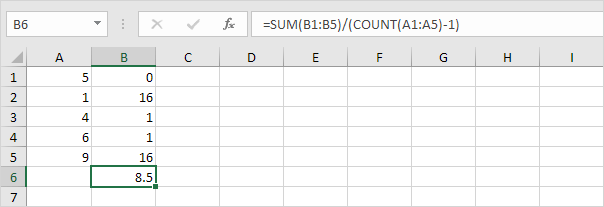2- Calculate the square root of the obtained number.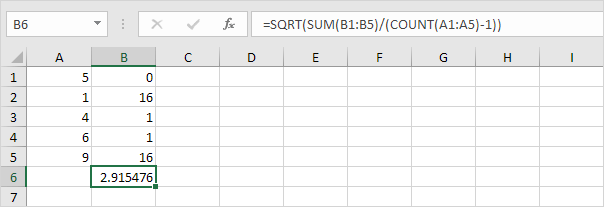3- Fortunately, the STDEV.S function in Excel can do all these steps for you.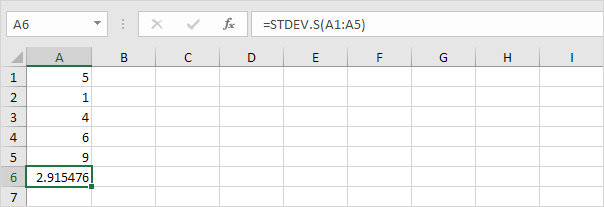Note: Why do we divide n by 1 instead of n when estimating the standard deviation based on the sample? In Bessel modification, dividing by n-1 instead of n gives us a better estimate of the standard deviation.